Unit Weight Determination - Sand Replacement Method

Unit weight of soil is weight of soil sample to the volume of that sample. For soils, such as hard soil or granular soil, where core cutter method cannot be applied, sand replacement method is used for the determination of unit weight of soil in its natural state.For this test to perform we will be needing a calibrating sand which passes through 1 mm IS sieve and retained on 600 micron IS sieve.

First we determine bulk unit weight of this sand and then using that we will determine the bulk unit weight of soil sample taken from the field.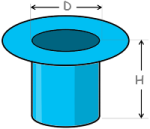Firstly we take a calibrating container and determine its volume by taking its measurements and doing some simple calculations.The volume of the calibrating container is roughly equal to 1000 cc (here cc is centimeter cube).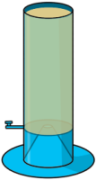Next we take a Sand pouring cylinder.

A sand pouring cylinder is a hollow cylinder which has cone type structure at the bottom. At the top of that cone there is a small hole so that sand to pass through it. Hole can be opened or closed using the knob provided.

Fill this sand pouring cylinder with the sand up to the top and take its weight to note down weight W1.

Then we put the sand pouring cylinder over the calibrating container and open the shutter so as to let the sand fall in and fill the container completely. Close the shutter and remove the cylinder.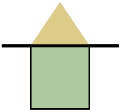Now take weight of this cylinder with the remaining soil and note down weight W2.

We will notice a cone type heap of sand has been made over the calibrating container.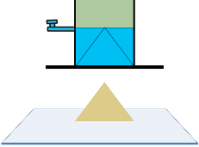To determine its weight we take a glass plate and put the sand pouring cylinder on it. Again we open its shutter and let the sand fill the conical part of the cylinder. When soil movement stops, close the shutter and remove the cylinder. Then weigh the soil on the glass plate and note the weight as W3.

Using these weights we can calculate how much soil was used to fill the volume of calibrating container.

We say this weight as WA, which can be written as W1 minus W2 minus W3.Hence bulk unit weight of the sand can be determined by its formula and will be equal to this.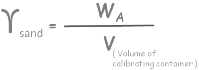Now come to field from where soil’s unit weight needs to be estimated.Clean and level a small area on the ground. Take a metal tray with central hole in the middle of it and place it on the cleaned surface.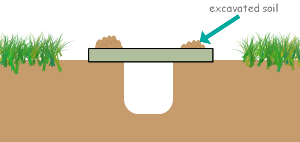Now excavate the ground from this hole using a knife up to roughly 15 centimeter and collect all the excavated soil into the tray.

After that collect all the excavated soil and take its weight. Note this weight as Wexcavated soil.

Next fill the sand pouring cylinder with the sand up to the top again. Weigh this cylinder and note down new WN1.

Then place this sand pouring cylinder over the excavated hole concentrically. Open the shutter and allow soil to fall into the hole and fill it completely. When no movement of sand is observed, close the shutter and remove the cylinder.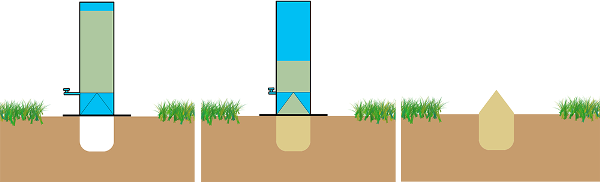Weigh this cylinder with remaining sand and note weight WN2.

Now if we remove the weight of cylinder with remaining soil and weight of conical heap of soil from weight of cylinder which is full of soil, we can determine the amount of sand is used to fill the hole, which we say as Wpoured soil.

Now we can calculate how much of sand is used to fill the hole by the simple calculation as WB equal to W1 minus W4 minus W2.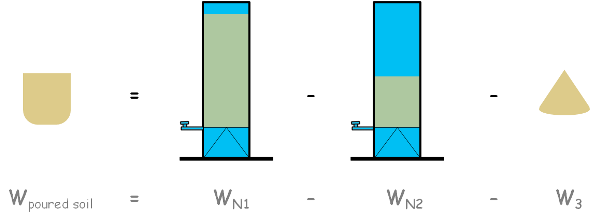Now here we know bulk unit weight of sand and weight of the sand which was used to fill the hole.

Using the bulk unit weight formula we can determine the volume of the hole as weight of sand used to fill the hole divided by unit weight of sand.Next now we know the weight of soil which we excavated from the hole and the volume of that hole. So again by simply applying bulk unit weight formula we can calculate the bulk unit weight of the soil we excavated.From this excavated soil if we take out a small sample and determine its water content using any of the water content determination method, we can calculate dry unit weight of soil using this formula.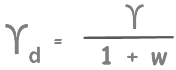Here gamma is bulk density what we have calculated here and w is water content.

Tags : Sand Replacement, unit weight, soil test

Published on :2019-09-06